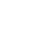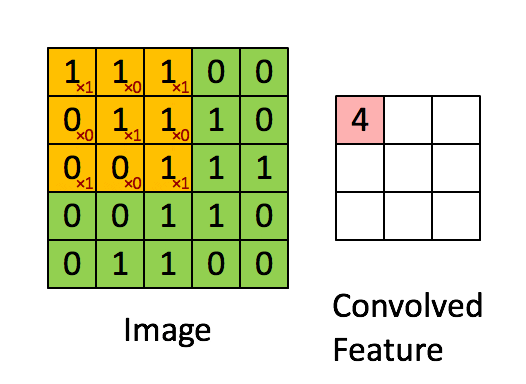# Understanding Convolutional Neural NetworksGuy Torbet

August 6, 2021

## What is a ConvNet

A lot of the problems that can benefit from convolutional nerual networks only use 2D-convolutions.

A convolution takes an N-by-N vector kernel, passes it through a filter, and outputs a K-by-K vector, where K<N.For all the nerds:orIntuitively, the matrix representation of the input vector is multiplied element-wise with the feature detector to produce a feature map, also known as a convolved feature or an activation map. The aim of this step is to reduce the size of the vector and make processing faster and easier. Some of the features of the matrix are lost in this step.

## Pooling

Spatial invariance is a concept where the location of an object in an image doesn’t affect the ability of the neural network to detect its specific features. Pooling enables the CNN to detect features in various images irrespective of the difference in lighting in the pictures and different angles of the images.

## Benefits

• They maintain translational invariance (in the paper, referred to as equivariance). When you translate the image or the filter, the cross-correlation of a filter with the image does not change.

• They are efficiently computable. If you fix the filter, then the convolution (series of multiply-adds) can be replaced with a FFT-multiply-IFFT. The overhead of the FFT/IFFT only makes sense if it can be amortized over many repeated convolutions with the same filter (see ).

• They are resource-efficient, especially when compared to a fully-connected layer. We impose the infinitely-strong prior of translational invariance, a symmetry which drastically cuts down the # of parameters we need.

I also have an email newsletter, it's about a 2-5 minute read every week of high quality tech and productivity content, you can sign up to it here!